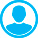ACCURACY ANALYSIS OF THE MODEL EXPERIMENTS ON DIPPING PURSE SEINE MODELS AT SIDE STREAMAbstract (English):
The research aim is to analyze accuracy of the experimental data obtained during the experiments in the hydraulic channel of "MariNPO", LLC (Kaliningrad) in 2014. During the experiments, three purse seine models were immersed under different loading of a leadline (0 kg, 0.248 kg, 0.338 kg) and different speed of the current (0.2 m/s, 0.3 m/s, 0.4 m/s). Seven experiments were carried out for each model (0 kg and 0.2 m/s; 0.248 kg and 0.2, 0.3, 0.4 m/s; 0.338 kg and 0.2, 0.3, 0.4 m/s). To confirm reliability of the data there was carried out the analysis of the total error which included: instrumental error, cargo error, measurement error of the net the models were made of, inaccuracy of immersion, displacement of the seine along OY axis, approximation error. To approximate the pilot data there was chosen the method of ordinary least squares. Approximation was conducted with a straight line (linear regression), polynomial n (polynomial regression), and a combination of arbitrary functions. The least error (6.82%) was obtained in the experiment 0 kg and 0.3 m/s, maximum (14.76%) - in the experiment 0.338 kg and 0.2 m/s. When the error doesn’t exceed 15%, subject to the adequate precision of measurement, the results of the experiments should be considered satisfactory.

Keywords:
purse seine, approximation, mathematical model